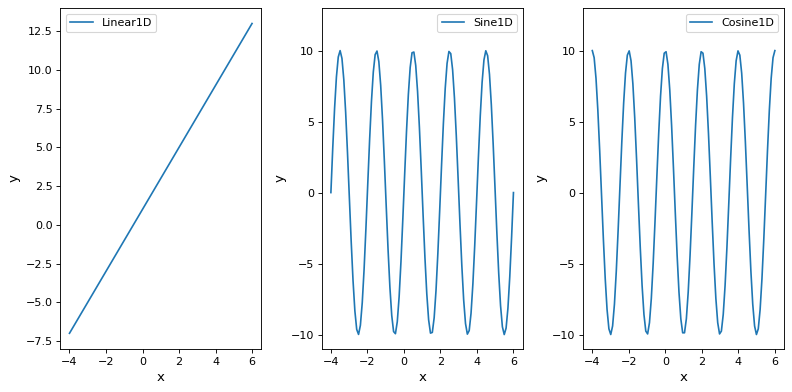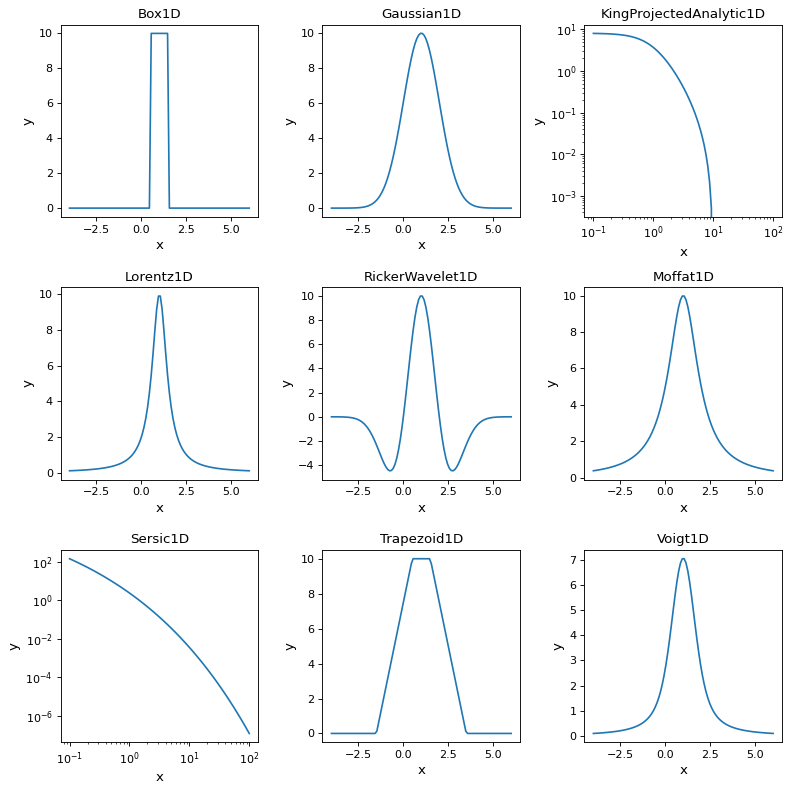1D Models¶

Operations¶

These models perform simple mathematical operations.

• Const1D model returns the constant replicated by the number of input x values.

• Multiply model multiples the input x values by a factor and propagates units if the factor is a Quantity.

• RedshiftScaleFactor model multiples the input x values by a (1 + z) factor.

• Scale model multiples by a factor without changing the units of the result.

• Shift model adds a constant to the input x values.

Shapes¶

These models provide shapes, often used to model general x, y data.

• Linear1D model provides a line parameterizied by the slope and y-intercept

• Sine1D model provides a sine parameterized by an amplitude, frequency, and phase.

• Cosine1D model provides a cosine parameterized by an amplitude, frequency, and phase.

(png, svg, pdf)Profiles¶

These models provide profiles, often used for lines in spectra.

• Box1D model computes a box function with an amplitude centered at x_0 with the specified width.

• Gaussian1D model computes a Gaussian with an amplitude centered at x_0 with the specified width.

• KingProjectedAnalytic1D model computes the analytic form of the a King model with an amplitude and core and tidal radii.

• Lorentz1D model computes a Lorentzian with an amplitude centered at x_0 with the specified width.

• RickerWavelet1D model computes a RickerWavelet function with an amplitude centered at x_0 with the specified width.

• Moffat1D model computes a Moffat function with an amplitude centered at x_0 with the specified width.

• Sersic1D model computes a Sersic model with an amplitude with an effective radius and the specified sersic index.

• Trapezoid1D model computes a box with sloping sides with an amplitude centered at x_0 with the specified width and sides with the specified slope.

• Voigt1D model computes a Voigt function with an amplitude centered at x_0 with the specified Lorentzian and Gaussian widths.

(png, svg, pdf)